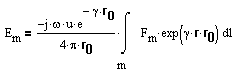# Source Code Description: Subroutine GFFLD

GFFLD

PURPOSE: to calculate the far-field for the thin wire structure.

METHOD: The far-field for the structure is calculated from the loop currents. The loop currents are either the currents produced by the transmitting antenna calculations of subroutine GANT1 or the currents produced by an incident plane wave.

In the incident field is generated by a distant source with spherical coordinates (r0, q0, f0), the excitation voltages induced by an incident plane wave arewhere

Ei = E0 exp(g r r0 ) dl

where E0 is a vector constant, r0 is a vector from the coordinate origin to the distant source, and r is the radial vector from the origin to the observation point.

The field Em is generated by test dipole m when radiating in the homogeneous medium. Using the vector potential, the field at the distant point (r0, q0, f0) iswhere the radial component is to be suppressed. From the above equations,If an antenna gain calculation is desired, INC is set to zero. PH and TH denote the spherical coordinate direction of the distant observation point. The phi-polarized (EPPS) and the theta-polarized (ETTS) components of the electric field intensity are returned to the calling program.

If INC = 1, a backscattering calculation is desired. In this case PH and TH denotes the incident angles for the incident plane wave. These are also the spherical coordinates of the distant source. The outputs returned to the calling program include absorption, extinction, and scattering cross section for each polarization: scattered electric field; and echo areas.

If INC = 2, a bistatic calculation is desired. In this case PH and TH denote the spherical coordinate of a distant observer. Since this calculation uses the induced loop currents (EP and ET), a backscattering call must proceed this calculation. The outputs returned to the calling program consist of the scattered electric field components and echo areas.

EPP(I) and ETT(I) denote the phi-polarized and theta-polarized far-zone fields of dipole mode I with unit terminal current. In a backscattering situation, the excitation voltages EP(I) and ET(I) are obtained by multiplying EPP and ETT by the constant CJI. Then calls are made to SQROT which stores the solution (the induced loop currents) in EP(I) and ET(I). RITE is called for the branch currents CG(J), and GDISS is called for the time-average power dissipated in the imperfectly conducting wire and the lumped loads. This power is denoted PDISS and TDISS for phi-polarized and theta-polarized incident waves, respectively.

In scattering problems, the incident plane wave has unit electric field intensity at the origin. GGG denotes the time-average power density of the incident wave at the origin. ACSP and ACST denote the absorption cross sections for the phi and theta polarizations.

PIN and TIN denote the time-average power input to the wire structure, delivered by the equivalent voltage generators VP and VT at the terminals, PIN and TIN apply for the phi and theta polarizations, respectivity. The time-average power input is regarded as the sum of the time-average power dissipated and the time-average power radiated or scattered by the wire. ECSP and ECST denote the extinction cross sections and SCSP and SCST denote the scattering cross sections.

The distant field is calculated in the DO LOOP ending with statement 7 for scattering situations, and in the DO LOOP ending with statement 9 for the antenna situation.

The radar cross sections (echo areas) SPPM, SPTM, STPM,and STTM are defined aswhere Ss and Si denote the time-average power densities in the scattered and incident fields evaluated at the origin.

For an antenna, the following definition is employed for the power gains:where Pi, GG, denote the time-average power input and S(r, q, f) is the time-average power density in the radiated field. GPP and GTT denote the power gains associated with the phi-polarized and the theta-polarized components of the field, respectively.

The use of the variables JFLAG and KPLAG are described in subroutine SGANT.

CALLED BY:

CALLS TO: